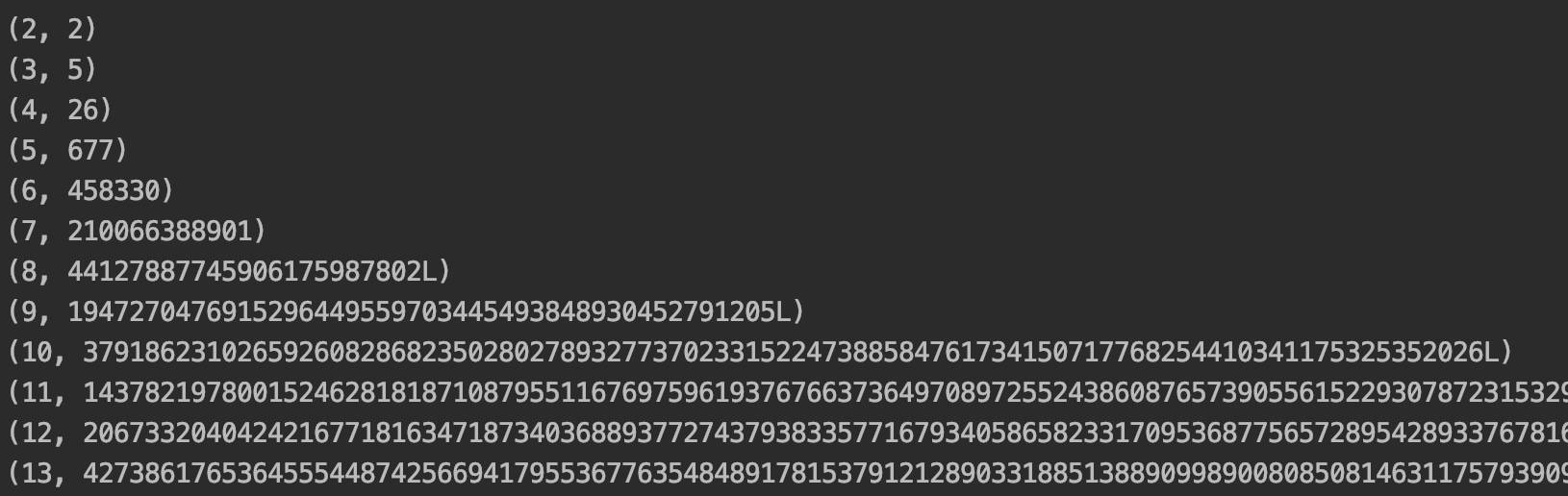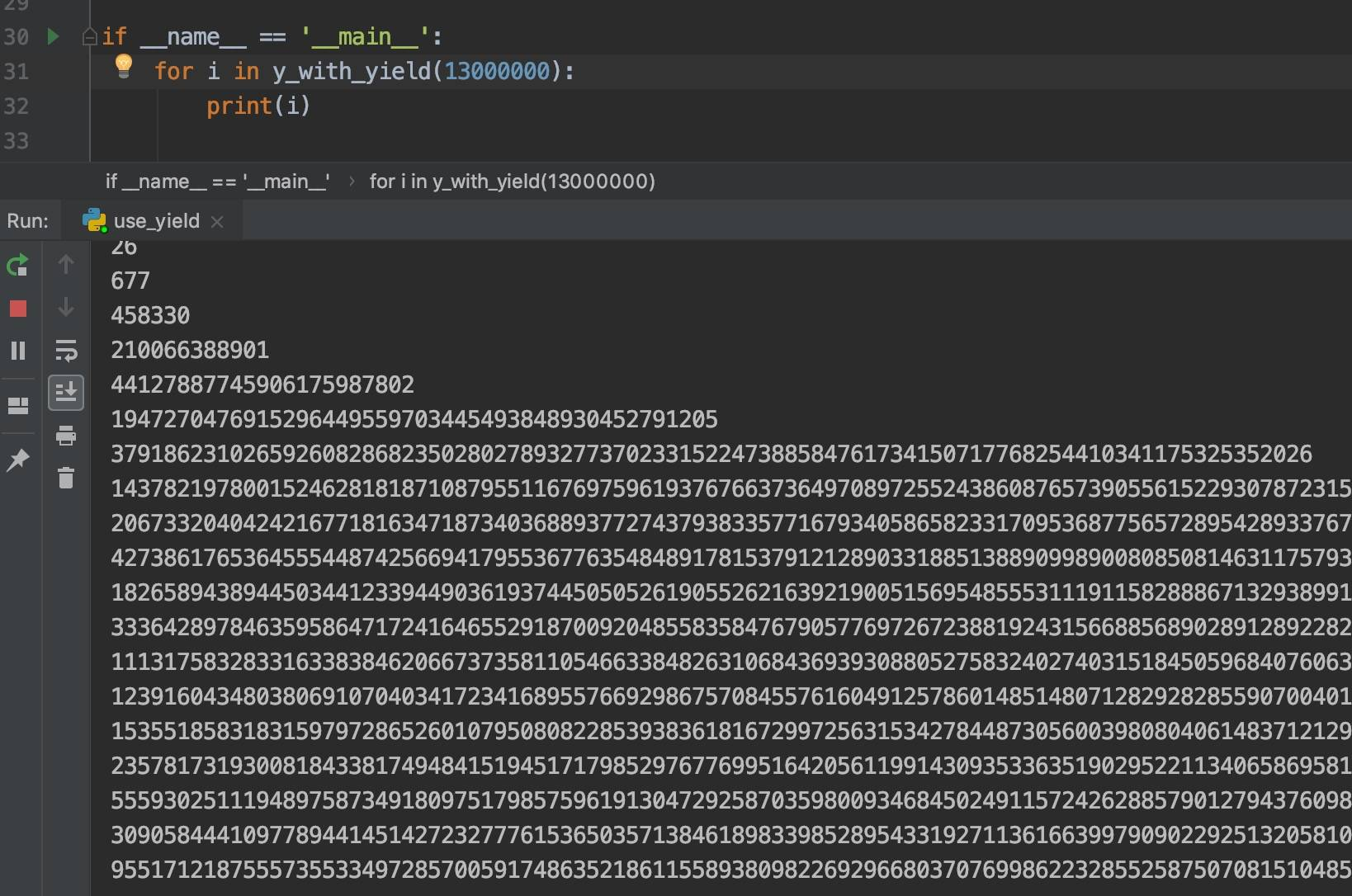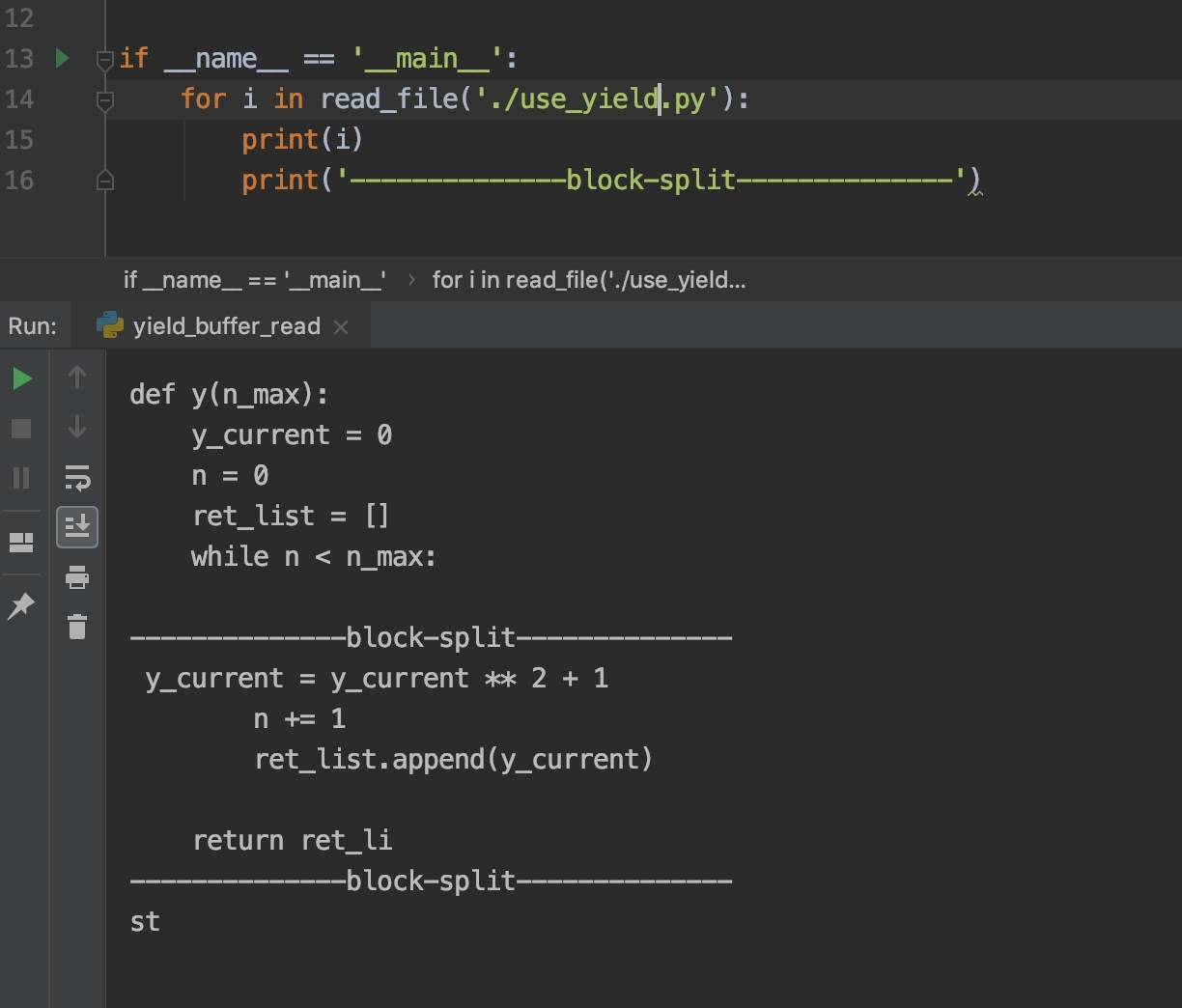# 《Python有什么好学的》之生成器/迭代器

“Python有什么好学的”这句话可不是反问句，而是问句哦。

## 为什么是斐波那契

``````斐波那契数列，数学表示为a(1)=0, a(2)=1, a(i)=a(i-1)+a(i-2) (i>=3)：
0 1 1 2 3 5 8 13 21 ...

``````

## 原始低效的循环

``````def y():
y_current = 1
n = 1
while n < 13:
y_current = y_current ** 2 + 1
n += 1
print(n, y_current)
``````1. 暴露n的值，n值可以作为参数输入，即我需要控制数列计算到哪
2. 函数返回（输出）整个数列

``````def y(n_max):
y_current = 0
n = 0
ret_list = []
while n < n_max:
y_current = y_current ** 2 + 1
n += 1
ret_list.append(y_current)

return ret_list

if __name__ == '__main__':
for i in y(13):
print(i)
``````

``````if __name__ == '__main__':
for i in y(13000000):
print(i)
``````## 生成器的小试牛刀

``````def y_with_yield(n_max):
y_current = 0
n = 0
while n < n_max:
y_current = y_current ** 2 + 1
n += 1
yield y_current

if __name__ == '__main__':
for i in y_with_yield(13000000):
print(i)
``````1. `for i in y_with_yield(13000000)`的循环中，每一次循环程序才会进入函数去计算，而不会把全部结果都计算出来再返回
2. 由于不会把全部结果都计算出来再返回，程序运行所需的内存也大幅地减少

1. 这个小生成器在较大数据的计算量时，有较大的优势
2. 程序把推导式的计算通过yield分散了，降低了cpu和内存的压力
3. 如果没有煎鱼的需求，这一切都白搭且多余

## 通过缓存机制读取文件

``````def read_file(file_path):

BLOCK_SIZE = 100
with open(file_path, 'rb') as f:
while True:
if block:
yield block
else:
return

if __name__ == '__main__':
print(i)
print('--------------block-split--------------')
``````## 生成器类和生成器对象

``````class Y(object):

def __init__(self, n_max):
self.n_max = n_max
self.n = 0
self.y = 0

def __iter__(self):
return self

def next(self):
if self.n < self.n_max:
self.y = self.y ** 2 + 1
self.n += 1
return self.y
raise StopIteration()

if __name__ == '__main__':
y = Y(13)
for i in y:
print(i)
``````

1. 类需要包含`__iter__()`函数，而返回的不一定是self，但是需要生成器
2. 实现next方法，“迭代”的逻辑请放到该函数里面
3. 如果需要引入别的库或者写别的函数，可以在类中随便加

``````class SuperY(object):

def __init__(self, n_max):
self.n_max = n_max

def __iter__(self):
return Y(self.n_max)

if __name__ == '__main__':
sy = SuperY(13)
for i in sy:
print(i)
``````

## 生成器的照妖镜

1. isgeneratorfunction()，字面意思，是否生成器函数
2. isgenerator()，还是字面意思，是否生成器
3. isinstance()，这个指某对象是否为某个类的实例

``````from use_yield import y_with_yield as y
from iter_obj import Y
from inspect import isgeneratorfunction, isgenerator
from types import GeneratorType
from collections import Iterator

if __name__ == '__main__':
print(isgeneratorfunction(y))  # True
print(isgeneratorfunction(Y))  # False
print(isgeneratorfunction(y(5)))  # False
print(isgeneratorfunction(Y(5)))  # False
print(isgenerator(y))  # False
print(isgenerator(Y))  # False
print(isgenerator(y(5)))  # True
print(isgenerator(Y(5)))  # False

print("")

print(isinstance(y, GeneratorType))  # False
print(isinstance(y(5), GeneratorType))  # True
print(isinstance(Y, GeneratorType))  # False
print(isinstance(Y(5), GeneratorType))  # False

print("")

print(isinstance(y, Iterator))  # False
print(isinstance(y(5), Iterator))  # True
print(isinstance(Y, Iterator))  # False
print(isinstance(Y(5), Iterator))  # True
``````

1. 带yield的y函数是一个生成器函数，带yield的y函数带上参数5后，称为了生成器。因为y是函数的引用，而带上了参数5后，y(5)就不再是函数了，而是通过yield进化成了生成器。
2. 生成器是类GeneratorType的实例，但很遗憾的是，生成器类的实例不是生成器（黑人问号）。
3. 然而，生成器y(5)和生成器类的实例都属于迭代器。

## 其他乱七八糟的

Python里面，range和xrange有什么不同，用哪个更好，为什么？

Categories: Python